“All in Cloud”之后，和你聊聊「云原生DevOps的Kubernetes技巧」 了解详情

# 使用开源概率编程语言 Pyro 对截尾时间 - 事件数据进行建模

• 2019 年 6 月 08 日
• 本文字数：5588 字

阅读完需：约 18 分钟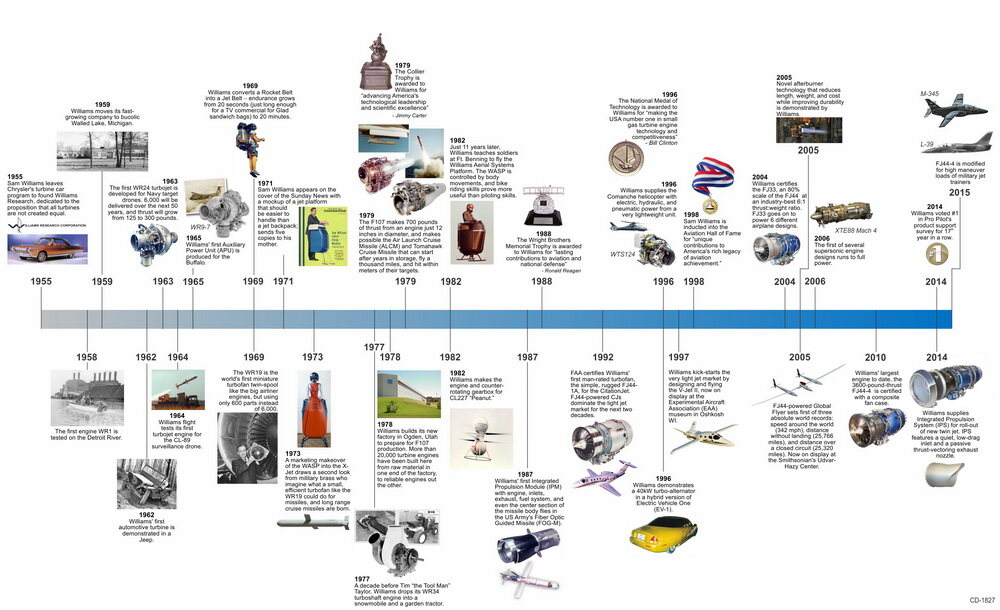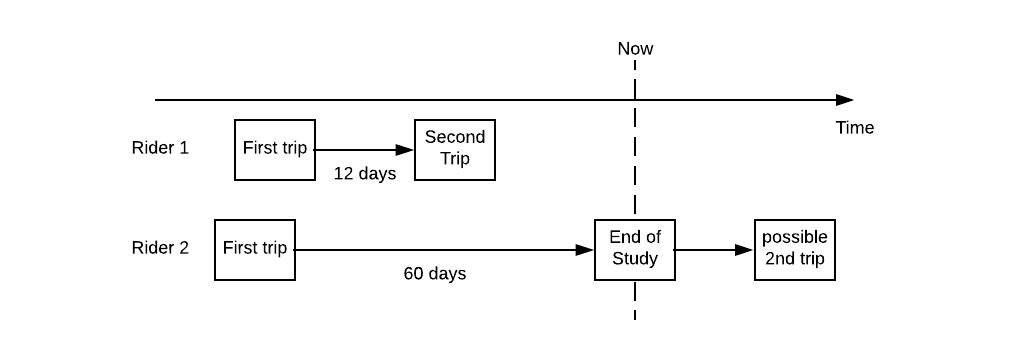## 用于统计建模的 Pyro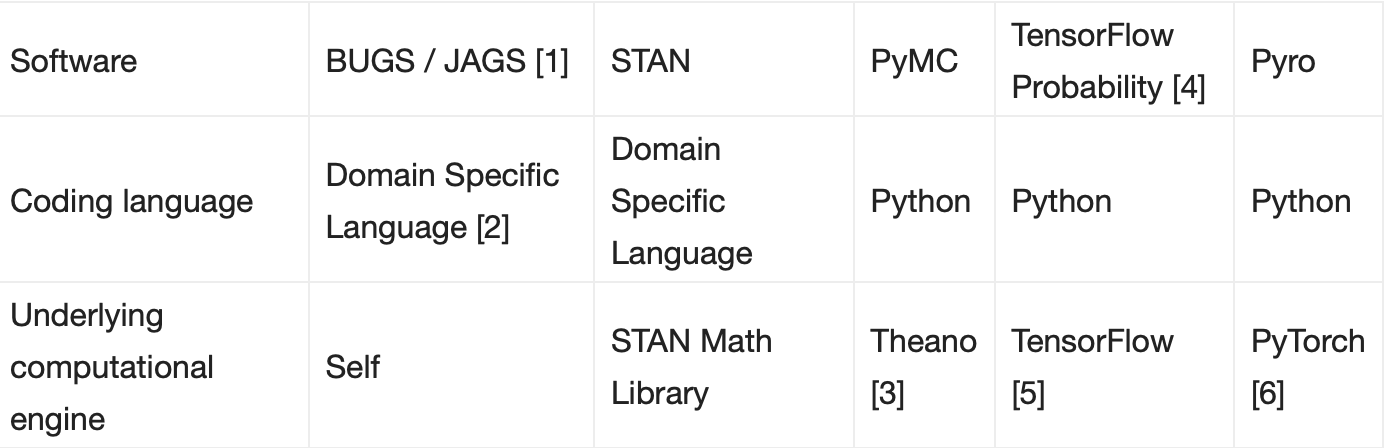1. BUGS/JAGS 是概率编程早期的例子。在统计领域，它们已经被积极开发和使用了 20 多年。

2. 但是，BUGS/JAGS 主要是从头设计和开发的。因此，模型规范是用它们特定于域的语言完成的。此外，概率程序开发人员需要从 R 和 MATLAB 中的包装器中调用 BUGS/JAGS。用户必须在编码语言和文件之间来回切换，不太方便。

3. PyMC 依赖于 Theano 后端。但是，Theano 项目最近停止了。

4. TensorFlow Probability（TFP）最初作为一个名为 Edward 的项目启动。该 Edward 项目已纳入 TFP 项目。

5. TFP 使用 TensorFlow 作为其计算引擎。因此，其仅支持静态计算图。

6. Pyro 使用 PyTorch 作为计算引擎，因此支持动态计算图。这使得用户能够在数据流方面指定不同的模型，非常灵活。

## 对截尾的时间-事件数据建模

### 模型定义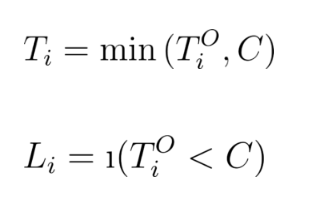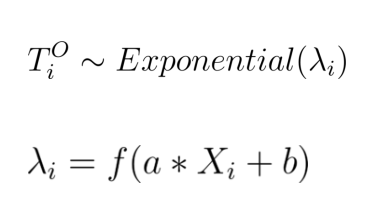### 生成人工数据

import pyro import torch import seaborn as sns import pyro.distributions as dist from pyro import infer, optimfrom pyro.infer.mcmc import HMC, MCMCfrom pyro.infer import EmpiricalMarginalassert pyro.__version__.startswith('0.3')

n = 500a = 2b = 4c = 8x = dist.Normal(0, 0.34).sample((n,)) # Note link = torch.nn.functional.softplus(torch.tensor(a*x + b))# note below, param is rate, not meany = dist.Exponential(rate=1 / link).sample()truncation_label = (y > c).float()y_obs = y.clamp(max=c)sns.regplot(x.numpy(), y.numpy())sns.regplot(x.numpy(), y_obs.numpy()) ## Note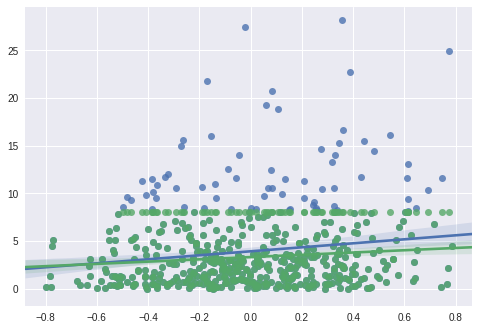### 构建模型

def model(x, y, truncation_label): ## Note    a_model = pyro.sample("a_model", dist.Normal(0, 10)) ## Note    b_model = pyro.sample("b_model", dist.Normal(0, 10))    link = torch.nn.functional.softplus(a_model * x + b_model) ## Note      for i in range(len(x)):    y_hidden_dist = dist.Exponential(1 / link[i]) ## Note          if truncation_label[i] == 0:       ## Note        y_real = pyro.sample("obs_{}".format(i),                            y_hidden_dist,                           obs = y[i])    else:      ## Note       truncation_prob = 1 - y_hidden_dist.cdf(y[i])      pyro.sample("truncation_label_{}".format(i),                   dist.Bernoulli(truncation_prob),                   obs = truncation_label[i])

• Note 1：总的来说，模型函数描述的是数据生成的过程。这个示例模型函数告诉我们如何从输入的矢量 x 生成 y 或 truncation_label。

• Note 2：我们指定这里﻿和﻿的先验分布，并利用 pyro.sample 函数对它们采样。Pyro 在 PyTorch 项目和 Pyro 项目中都有大量的随机分布。

• Note 3: 在这里，我们把输入﻿，﻿和﻿接入用变量 link 表示的﻿矢量。

• Note 4：我们利用带有尺度参数矢量链接的指数分布来指定真实时间-事件﻿的分布。

• Note 5：对于观察 i，如果我们观察到时间-事件数据，那么我们把它和实际观察 y[i]进行对比。

• Note 6：如果对于观察﻿，数据是截尾的，那么截断标签（这里等于 1）遵循伯努利分布。在﻿点，观察到截断数据的概率是﻿的 CDF。我们从伯努利分布中采样，并将其与 truncation_label[i]的实际观察结果进行对比。

### 用哈密顿•蒙特•卡罗方法（Hamiltonian Monte Carlo，简称 HMC）计算推理

pyro.clear_param_store()# note  hmc_kernel = HMC(model,                 step_size = 0.1,                  num_steps = 4)# Note  mcmc_run = MCMC(hmc_kernel,                 num_samples=5,                warmup_steps=1).run(x, y, truncation_label)# Note  marginal_a = EmpiricalMarginal(mcmc_run,                                sites="a_model")# Note  posterior_a = [marginal_a.sample() for i in range(50)] sns.distplot(posterior_a)

def model(x, y, truncation_label):  a_model = pyro.sample("a_model", dist.Normal(0, 10))   b_model = pyro.sample("b_model", dist.Normal(0, 10))    link = torch.nn.functional.softplus(a_model * x + b_model)     with pyro.plate("data"):    y_hidden_dist = dist.Exponential(1 / link)         with pyro.poutine.mask(mask = (truncation_label == 0)):       pyro.sample("obs", y_hidden_dist,                  obs = y)          with pyro.poutine.mask(mask = (truncation_label == 1)):      truncation_prob = 1 - y_hidden_dist.cdf(y)      pyro.sample("truncation_label",                   dist.Bernoulli(truncation_prob),                   obs = torch.tensor(1.))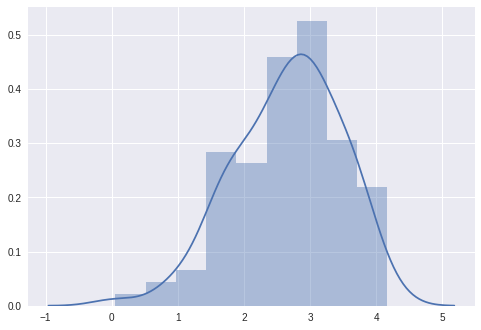### 利用变分推理加速估计

guide = AutoMultivariateNormal(model)

### 训练模型并推断结果

pyro.clear_param_store()  adam_params = {"lr": 0.01, "betas": (0.90, 0.999)}optimizer = optim.Adam(adam_params)svi = infer.SVI(model,                 guide,                 optimizer,                 loss=infer.Trace_ELBO())losses = []for i in range(5000):  loss = svi.step(x, y_obs, truncation_label)  losses.append(loss)  if i % 1000 == 0:    print(', '.join(['{} = {}'.format(*kv)                     for kv in guide.median().items()]))print('final result:')for kv in sorted(guide.median().items()):  print('median {} = {}'.format(*kv))

a_model = 0.009999999776482582, b_model = 0.009999999776482582a_model = 0.8184720873832703, b_model = 2.8127853870391846a_model = 1.3366154432296753, b_model = 3.5597035884857178a_model = 1.7028049230575562, b_model = 3.860581874847412a_model = 1.9031578302383423, b_model = 3.9552347660064697final result:median a_model = 1.9155923128128052median b_model = 3.9299516677856445

sns.plt.plot(losses)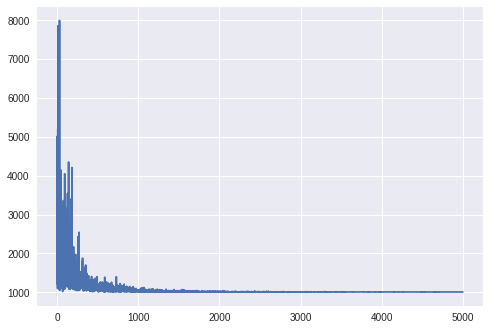N = 1000for name, quantiles in guide.quantiles(torch.arange(0., N) / N).items():  quantiles = np.array(quantiles)  pdf = 1 / (quantiles[1:] - quantiles[:-1]) / N  x = (quantiles[1:] + quantiles[:-1]) / 2  sns.plt.plot(x, pdf, label=name)  sns.plt.legend()sns.plt.ylabel('density')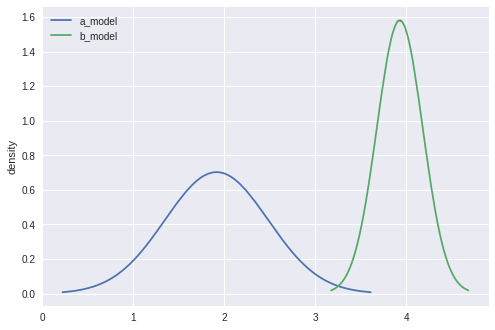## 其他

2019 年 6 月 08 日 08:006487## 评论

本文来自华为云开发者社区

• ###### 容量预测（上）：第三只眼，通过 AI 预测服务容量瓶颈

基于AI进行容量预测要经过特征选取、建立模型、准确度评价三大步骤

2021 年 5 月 28 日

• ###### 谷歌 MetNet：秒级别预测的神经网络天气模型

整个预测耗时只需几秒钟，之前的模型需要1小时

• ###### 41 丨数据挖掘实战（3）：如何对比特币走势进行预测？

今天我带你用数据挖掘对比特币的走势进行预测和分析，采用哪种方法比较好呢？

2019 年 3 月 18 日

• ###### 概率和统计：编程为什么需要概率和统计？

我们需要掌握哪些概率统计知识？这些知识可以用在什么地方？第二模块第一节，我们就来聊聊这些问题。

2019 年 1 月 28 日

• ###### 为什么我的模型表现这么差？

阅读本文，你将学到如何识别这些现象的必要的知识，并获得如何克服这些现象的工具和补救措施，最终提高你的模型性能，这是每一个机器学习工程师的真正目标。

• ###### 宣布推出开源时间序列建模工具包 Gluon Time Series

今天，我们宣布推出 Gluon Time Series (GluonTS)，这是一种使用 Gluon API 的 MXNet 时间序列分析工具包。

• ###### 统计基础（上）：系统掌握指标的统计属性

在学习、解决技术问题的时候，我们都知道有这么一句话“知其然知其所以然”。那么，A/B测试的“所以然”是什么呢？

2020 年 12 月 2 日

• ###### 可视化方法对机器学习至关重要（系列）之一

Python和high level的机器学习/深度学习库，比如Scikit-learn，TensorFlow，NLTK，PyBrain，Theano和MLPY让机器学习走进“大众”（开发社区）视野。随着这些工具的开源，现在有了越来越多的机器学习从业者。与此同时，机器学习的份额并没有增加。预测工具正在成为各行各业（从商业，艺术，和工程到教育，法律和国防）的决策驱动。

• ###### 构建贝叶斯深度学习分类器

最近贝叶斯深度学习变得越来越流行，并且正在开发新技术在模型中引入不确定性，同时参数量与传统模型相同。

• ###### 统计分析 S 语言的优点和发展背景

S语言是由贝尔实验室开发的一种用来进行数据探索、统计分析、作图的解释型语言。它的丰富的数据类型，特别有利于实现新的统计算法，其交互式运行方式及强大的图形及交互图形功能使得我们可以方便的探索数据。资深分析师Catherine最近撰文详细介绍了S语言的优点和背后的发展历史。

• ###### 超级碗历史数据集分析（1967~2020 年）

看看作者是怎么对超级碗历史数据集进行分析的，相信对你一定有所裨益。

• ###### 导读：如何在机器学习中运用线性代数工具？

通过一个简单机器学习算法，讲解机器学习背后的线性代数真相，让你对线性代数有一个感性的认知。

2020 年 7 月 27 日

• ###### 如果关注排序效果，那么这个模型可以帮到你

矩阵分解在推荐系统中的地位非常崇高，恐怕本专栏介绍的其他算法模型都不能轻易地撼动它。

2018 年 3 月 30 日

• ###### 机器学习技术之预测性维护

在此文中，作者们探讨了如何构建机器学习模型进行系统的预测性维护。他们讨论了一个应用例子，该例子使用NASA的引擎故障数据集，用回归模型来预测引擎的剩余使用寿命。

• ###### Python 数据可视化的 10 种技能

今天我来给你讲讲Python的可视化技术。

• ###### 吴恩达团队提出倒计时回归模型：用 AI 技术预测病患死亡时间

近日，斯坦福大学Andrew Ng团队开发出了一种使用人工智能来预测病人死亡时间的系统。科学家希望该系统可以为病人提供更好的临床关怀。作者提出了一种新的预报检验方法Survival-CRPS，通过优化连续分级概率评分来提高预报的锐度，同时保持预报的校准度。这是科学界首次将最大似然法之外的评分方法成功应用于大型生存预测任务。

• ###### 机器学习在高德地图轨迹分类的探索和应用

本文从给予概率密度分布的贝叶斯模型视角与基于轨迹点图像编码的深度学习视角分别探索了轨迹分类可能的技术方案。

28天写作

28天写作

#### 【Mysql-InnoDB 系列】事务模型